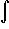#Interactive Real Analysis

Next | Previous | Glossary | Map

## 7.4. Lebesgue Integral

### Example 7.4.11(a): Properties of the Lebesgue Integral

Is the function f(x) = x ex Lebesgue integrable over the Cantor middle-third set? If so, find the integral.
Define the function g(x) = 0 on [0, 1]. Then f = g except on the Cantor middle third set C. Since m(C) = 0 the functions f and g agree except on a set with measure zero.

Therefore the integrals of f and g also agree, but clearly[0, 1] g(x) dx = 0
so thatC f(x) dx = 0
as well

We may have skipped a step to go from the integral over [0, 1] to the integral over C, but the details are easily filled in.

Next | Previous | Glossary | Map